# Digital Electronics - Combinational Logic Analysis

### Exercise :: Combinational Logic Analysis - General Questions

16.

A 4-variable AND-OR circuit produces a 0 at its Y output. Which combination of inputs is correct?

 A. A = 0, B = 0, C = 1, D = 1 B. A = 1, B = 1, C = 0, D = 0 C. A = 1, B = 1, C = 1, D = 1 D. A = 1, B = 0, C = 1, D = 0

Answer: Option D

Explanation:

No answer description available for this question. Let us discuss.

17.

A 4-variable AND-OR circuit produces a 1 at its Y output. Which combination of inputs is correct?

 A. A = 0, B = 0, C = 0, D = 0 B. A = 0, B = 1, C = 1, D = 0 C. A = 1, B = 1, C = 0, D = 0 D. A = 1, B = 0, C = 0, D = 0

Answer: Option C

Explanation:

No answer description available for this question. Let us discuss.

18.

Implementing the expression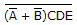using NAND logic, we get: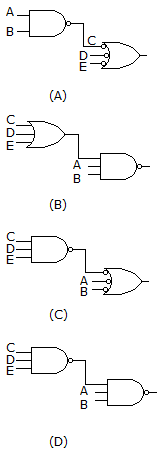A. (A) B. (B) C. (C) D. (D)

Answer: Option D

Explanation:

No answer description available for this question. Let us discuss.

19.

Implementing the expression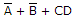using NAND logic, we get: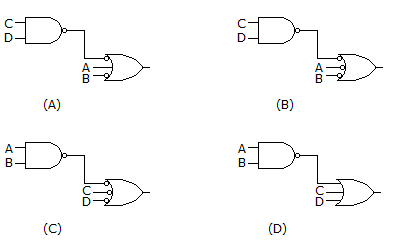A. (A) B. (B) C. (C) D. (D)

Answer: Option B

Explanation:

No answer description available for this question. Let us discuss.

20.

The following waveform pattern is for a(n) ________.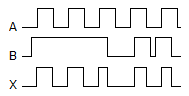A. 2-input AND gate B. 2-input OR gate C. Exclusive-OR gate D. None of the above

Answer: Option A

Explanation:

No answer description available for this question. Let us discuss.

#### Current Affairs 2021

Interview Questions and Answers